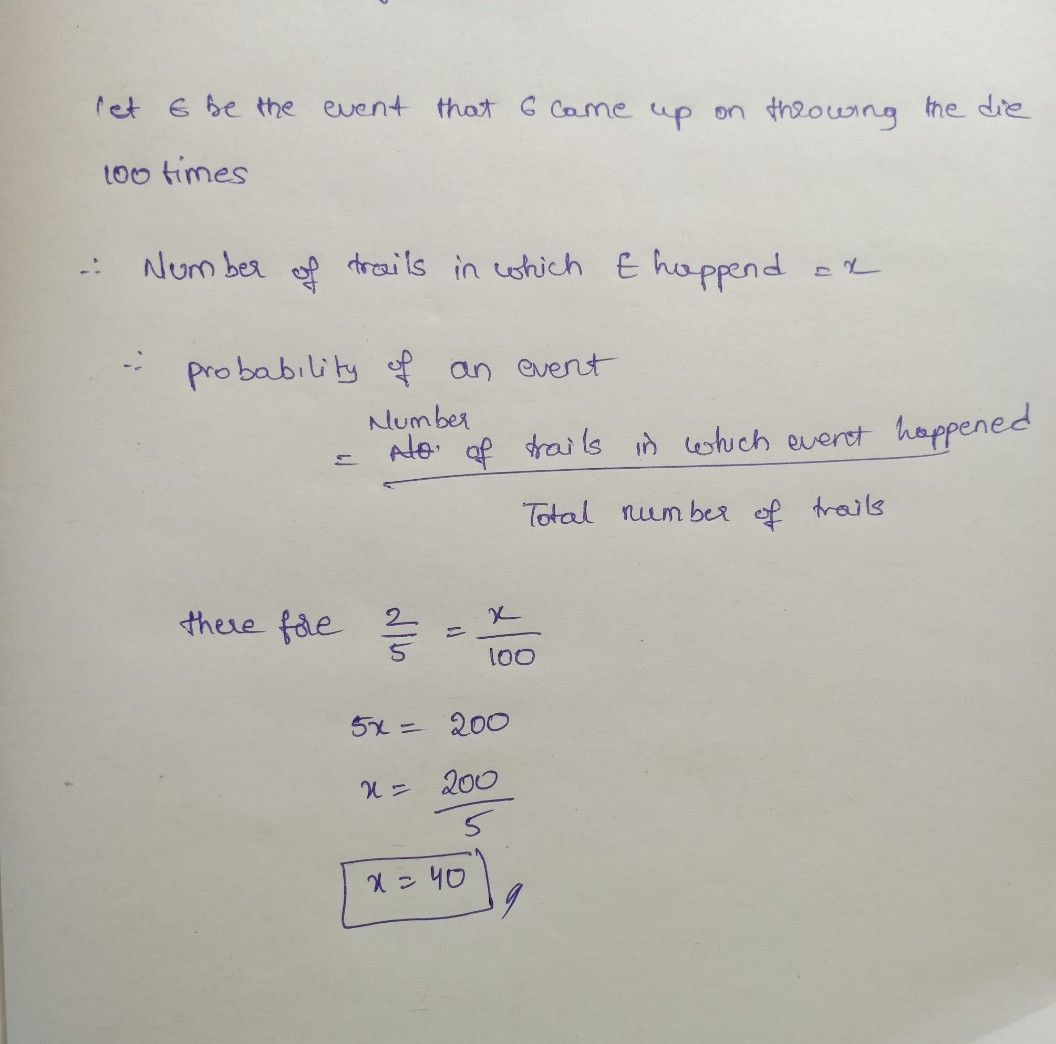Symbol
Problem$y.$ $34.$ The die has been rolled for $100$ times, the number of times $6$ appeared $M$ noted. If the probability of $6$ appeared was $2f50$ how many times $6$ didn appear?